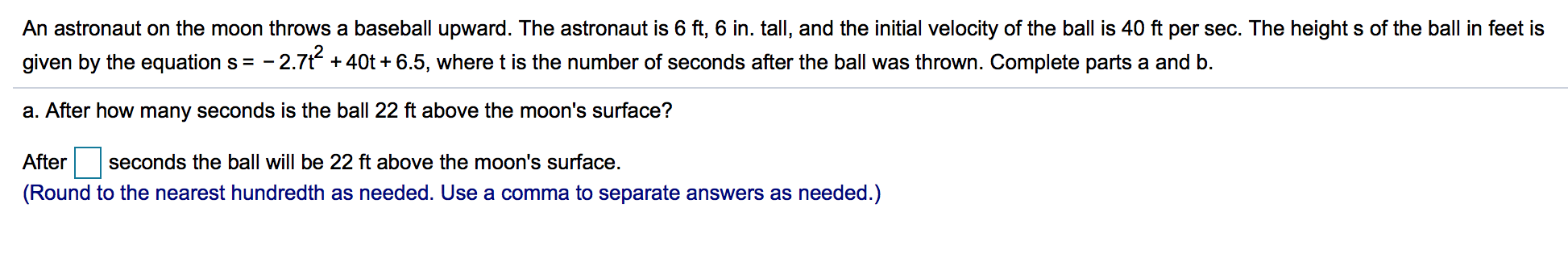# An astronaut on the moon throws a baseball upward. The astronaut is 6 ft, 6 in. tall, and the initial velocity of the ball is 40 ft per sec. The height s of the ball in feet isgiven by the equation s =-2.7t + 40t + 6.5, where t is the number of seconds after the ball was thrown. Complete parts a and b.a. After how many seconds is the ball 22 ft above the moon's surface?After seconds the ball will be 22 ft above the moon's surface.(Round to the nearest hundredth as needed. Use a comma to separate answers as needed.)2

Question
1808 viewshelp_outlineImage TranscriptioncloseAn astronaut on the moon throws a baseball upward. The astronaut is 6 ft, 6 in. tall, and the initial velocity of the ball is 40 ft per sec. The height s of the ball in feet is given by the equation s =-2.7t + 40t + 6.5, where t is the number of seconds after the ball was thrown. Complete parts a and b. a. After how many seconds is the ball 22 ft above the moon's surface? After seconds the ball will be 22 ft above the moon's surface. (Round to the nearest hundredth as needed. Use a comma to separate answers as needed.) 2 fullscreen
check_circle

Step 1

a) Calculating the time after which bal...

### Want to see the full answer?

See Solution

#### Want to see this answer and more?

Solutions are written by subject experts who are available 24/7. Questions are typically answered within 1 hour.*

See Solution
*Response times may vary by subject and question.
Tagged in

### Applications of Mathematics